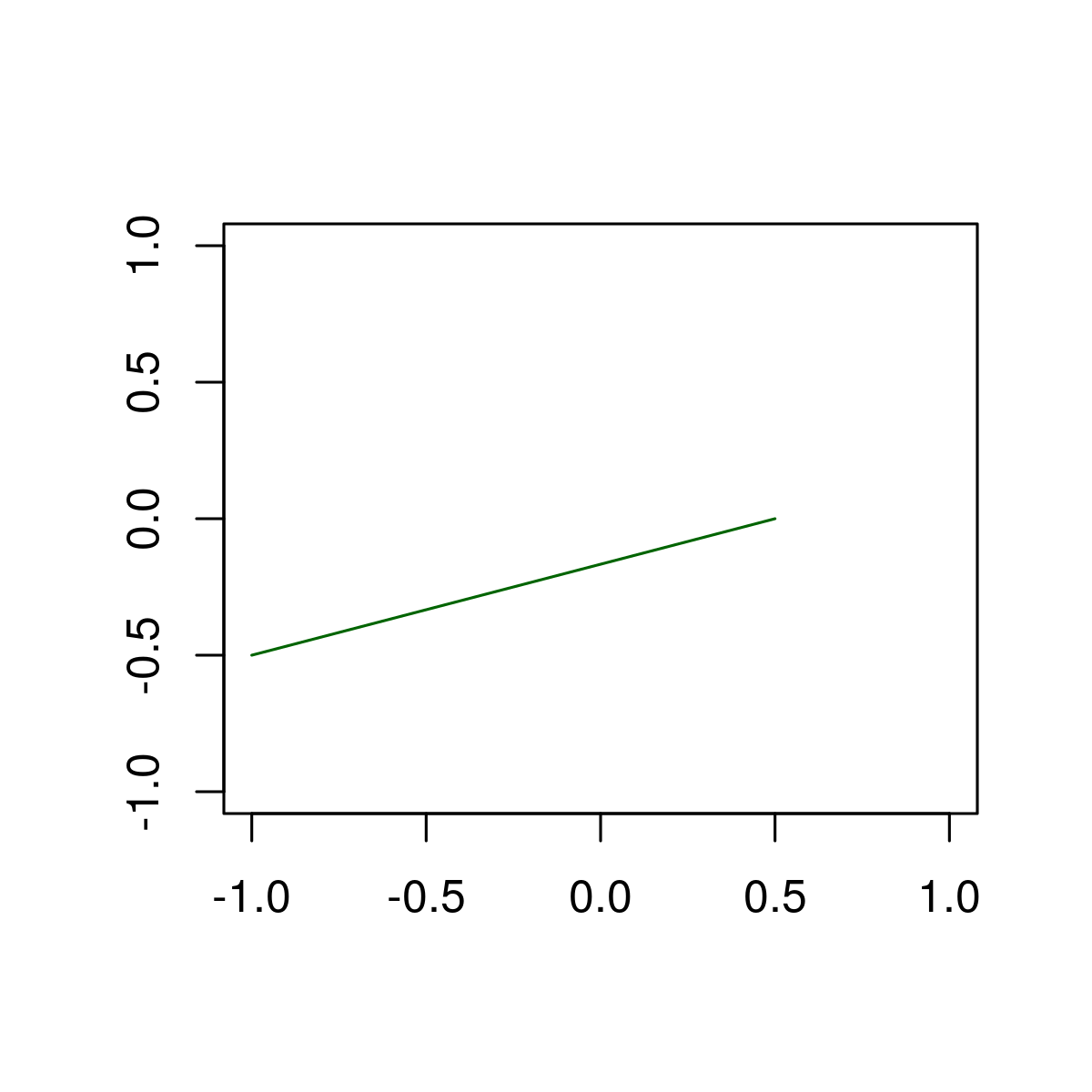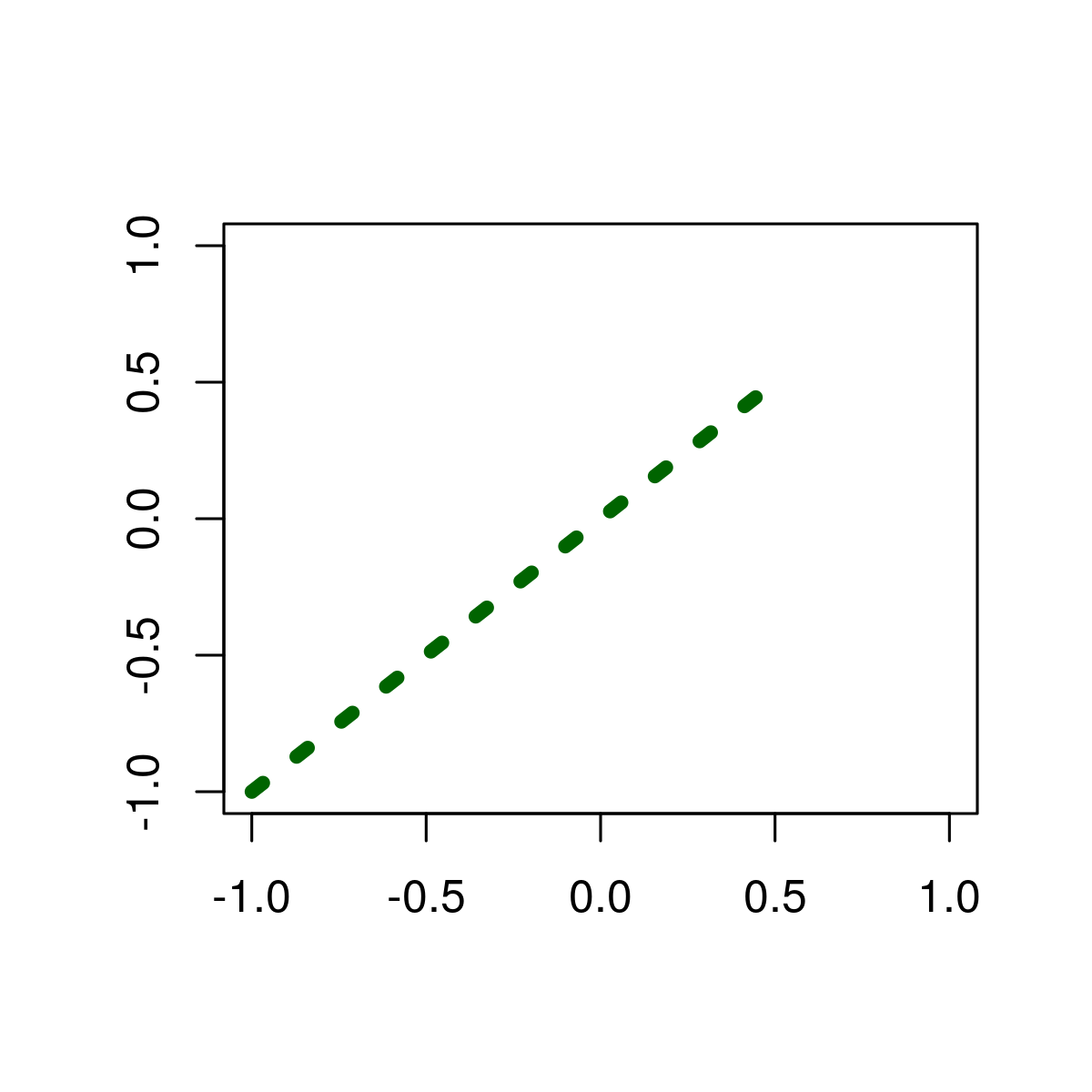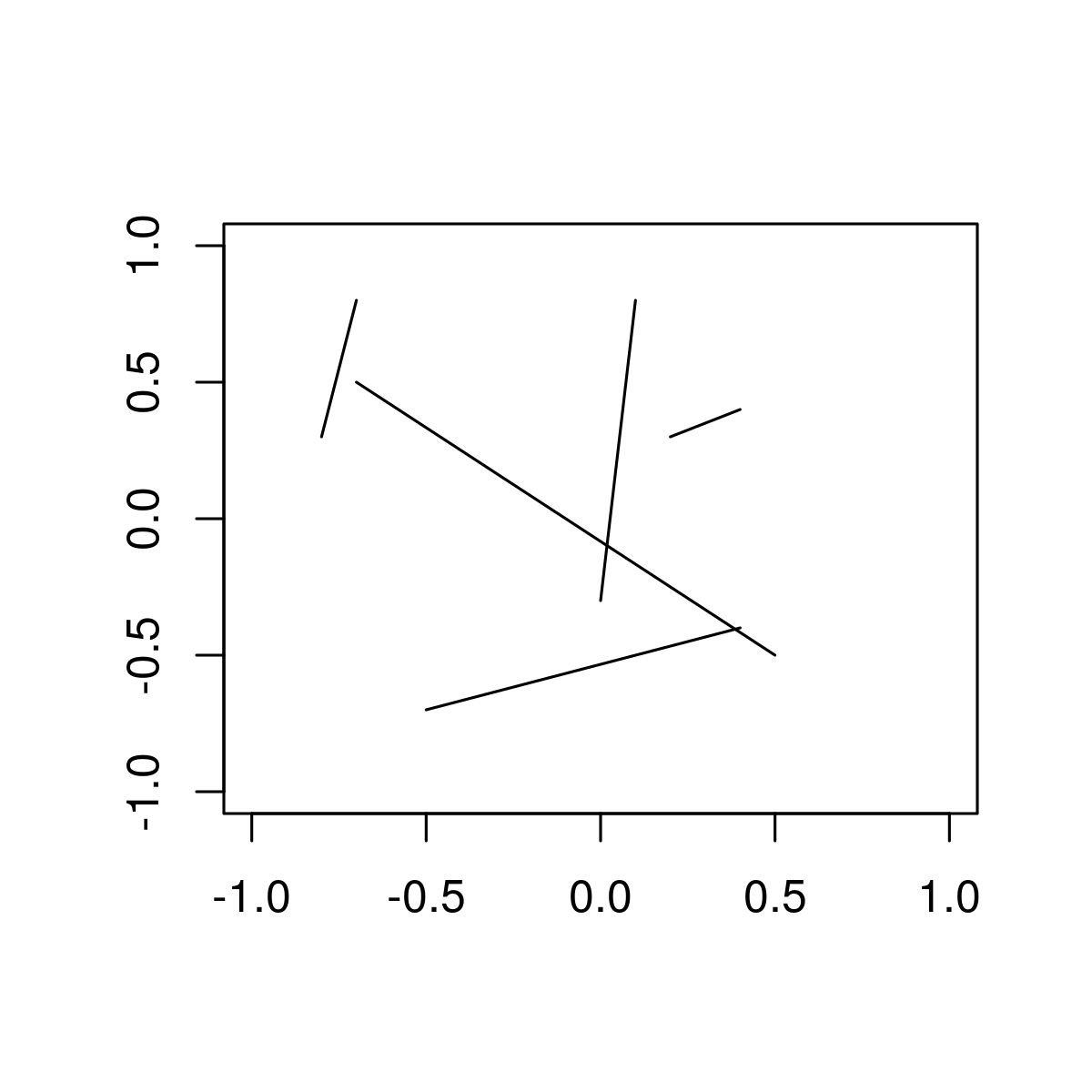# Draw Line Segments between Particular Points in R Programming – segments() Function

`segment()` function in R Language is used to draw a line segment between to particular points.

Syntax: segments(x0, y0, x1, y1)

Parameters:
x, y: coordinates to draw a line segment between provided points.

Returns: a line segment between given points

Example 1: To draw a single line segment

 `# Create empty example plot ` `plot``(0, 0, col = ``"white"``, xlab = ``""``, ylab = ``""``) ` ` `  `# Draw one line ` `segments``(x0 = - 1, y0 = - 0.5, x1 = 0.5, y1 = 0, col = ``"darkgreen"``)  `

Output:Here, x0 & y0 are starting points of the line segment and x1 & y1 are ending points of line segment .

Example 2: Modifying  color, thickness & line type

 `# Create empty example plot ` `plot``(0, 0, col = ``"white"``, xlab = ``""``, ylab = ``""``) ` ` `  `# Draw one line as in Example 1 ` `segments``(x0 = - 1, y0 = - 1, x1 = 0.5, y1 = 0.5, ` `          `  `         ``# Color of line ` `         ``col = ``"darkgreen"``,       ` `          `  `         ``# Thickness of line ` `         ``lwd = 5,                 ` `          `  `         ``# Line type ` `         ``lty = ``"dotted"``)                      `

Output:Example 3: Draw multiple line segments to R Plot.

 `# Create empty example plot ` `plot``(0, 0, col = ``"white"``, xlab = ``""``, ylab = ``""``)        ` ` `  `# Create data frame with line-values ` `multiple_segments <- ``data.frame``(x0 = ``c``(0.1, 0.2, - 0.7, 0.4, - 0.8),    ` `                       ``y0 = ``c``(0.8, 0.3, 0.5, - 0.4, 0.3), ` `                       ``x1 = ``c``(0, 0.4, 0.5, - 0.5, - 0.7), ` `                       ``y1 = ``c``(- 0.3, 0.4, - 0.5, - 0.7, 0.8)) ` `                        `  ` ``# Draw multiple lines                        ` ` ``segments``(x0 = multiple_segments\$x0,                    ` `         ``y0 = multiple_segments\$y0, ` `         ``x1 = multiple_segments\$x1, ` `         ``y1 = multiple_segments\$y1) `

Output:My Personal Notes arrow_drop_upCheck out this Author's contributed articles.

If you like GeeksforGeeks and would like to contribute, you can also write an article using contribute.geeksforgeeks.org or mail your article to contribute@geeksforgeeks.org. See your article appearing on the GeeksforGeeks main page and help other Geeks.

Please Improve this article if you find anything incorrect by clicking on the "Improve Article" button below.

Article Tags :

Be the First to upvote.

Please write to us at contribute@geeksforgeeks.org to report any issue with the above content.